Chapter 2.8, Problem 3E### Single Variable Calculus: Early Tr...

8th Edition
James Stewart
ISBN: 9781305270343

#### Solutions

Chapter
Section### Single Variable Calculus: Early Tr...

8th Edition
James Stewart
ISBN: 9781305270343
Textbook Problem

# Match the graph of each function in (a)–(d) with the graph of its derivative in I–IV. Give reasons for your choices.(a)(b)(c)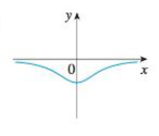(d)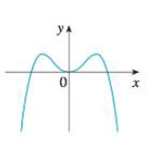I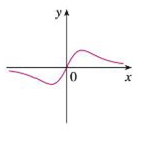IIIII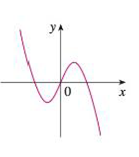IV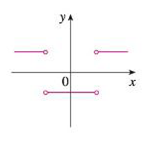(a)

To determine

To match: The graph of f with graph of its derivative with proper explanation.

Explanation

Given:

The given graph of the function f is shown in Figure 1.

Observation:

From the Figure 1, it is observed that the graph of f contains the horizontal tangents at two points. Let the two points be A and B.

Note that, the value of the derivative will be zero at the point where the function has the horizontal tangent...

(b)

To determine

To Match: The graph of of f with graph of its derivative with proper explanation.

(c)

To determine

To match: The graph of f with graph of its derivative with proper explanation.

(d)

To determine

To match: The graph of f with graph of its derivative with proper explanation.

### Still sussing out bartleby?

Check out a sample textbook solution.

See a sample solution

#### The Solution to Your Study Problems

Bartleby provides explanations to thousands of textbook problems written by our experts, many with advanced degrees!

Get Started

#### In Problems 1-6 simplify each fraction. 3.

Mathematical Applications for the Management, Life, and Social Sciences

#### Differentiate the function. F(z)=A+Bz+Cz2z2

Single Variable Calculus: Early Transcendentals

#### True or False: , for –π ≤ t ≤ π, is smooth.

Study Guide for Stewart's Multivariable Calculus, 8th